# Programming - Java Graph Eulerian Circuit Detection Algorithm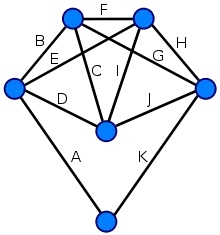Hello its a me again Drifter Programming. Today we continue with Java Graph Algorithms talking about how we check for Eulerian Circuits inside of a Graph. If you want to know about Hamiltonian Circuits then check out my post about that here. So, without further do, let's get straight into it!

## Eulerian Circuit Problem:

As we already know from previous time, a Hamiltonian Circuit is a path where we visit all the Vertices one time getting back to where we started. In the same way a Eulerian path is a path where we visit all the Edges one time. If we also get back to where we started, then this path is called a Eulerian Circuit/Cycle. A Graph that contains/has a Eulerian Circuit is called Eulerian and a graph that contains/has a Eulerian Path is called Semi-Eulerian.

This problem seems to be NP-Complete again (polynomial complexity) like the Hamiltonian Cycle problem, but it actually isn't! We can find out if a Graph is Eulerian, Semi-Eulerian or not in O(V+E) time, that is not polynomial!

So, the question now is: "How do we find out if a Graph is Eulerian or not?"

Thinking about the degree of a Vertex as the number of neighbours/Edges pointing out we find out that the following Properties apply for an Eulerian Path and an Eulerian Cycle respectively.

### Eulerian Path Properties:

• All vertices with non-zero degree are connected. (zero degree vertices don't belong to a Eulerian Path or Cycle)
• Up to 2 vertices have odd degree and all other vertices have even degree. (note that we can't have only one odd degree vertex, cause the sum of all degrees is always even in an undirected graph, and with 0 we end up with a Cycle)

### Eulerian Cycle Properties:

• All vertices with non-zero degree are connected. (same as in a path)
• All vertices have even degree.

A funny thing is that a graph with no edges is also considered Eulerian because we can't traverse through the edges :P :D

You might now wonder why these two properties (one being shared) must be and have to be true...

Well, every time we visit a vertex we go through two unvisited edges, where one has the currently visited vertex as an endpoint. For this to happen, the middle vertices in an Eulerian Path must have an even degree (2, 4 etc.). In an Eulerian Cycle any vertex can be a middle vertex and so all the vertices must have an even degree and none can have an odd degree.

## Examples:

Eulerian Path: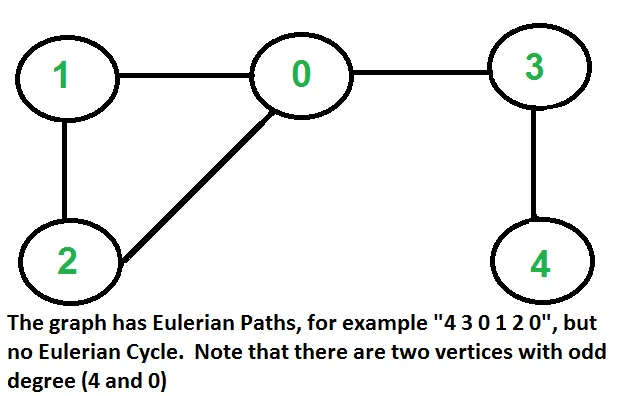Eulerian Cycle: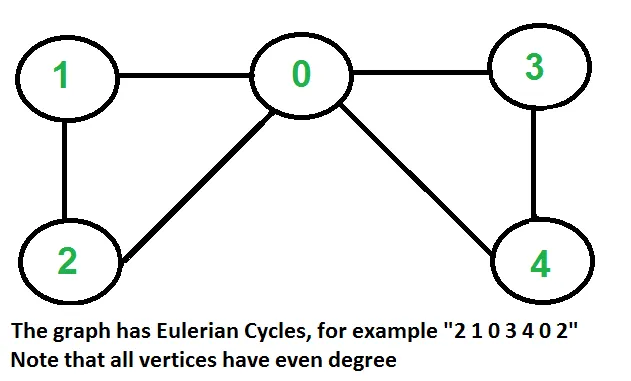## Algorithm:

The simplest algorithm we can think about will just check these properties to determine if the Graph contain a Eulerian Path, Cycle or nothing.

So, we will do the following steps:

1. check if all non-zero degree edges are connected (If at least one is not connected then the Graph doesn't contain an Eulerian Path or a Cycle)
2. count the number of odd-degree vertices (if 0 then Eulerian, if 2 then Semi-Eulerian, if >2 then non-Eulerian)

This can be easily implemented in Java as we will see in a bit...

## Algorithm Implementation in Java:

The algorithm and detection only works on undirected graphs and so we will use the Adjacency List implementation (for simpler counting of odd vertices) and tweak it to also add the opposite Edges to the Graph.

We have to use a Traversal algorithm to check if we can reach all the vertices starting from one vertex. For that Kosaraju's DS algorithm is recommended, but I will use a simple DFS Algorithm. You can find another implementation here in my post of some Months ago.

The Code looks like this:

Graph class: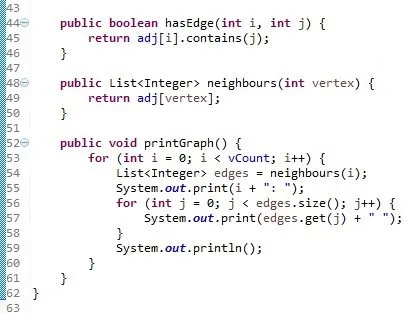Algorithm code: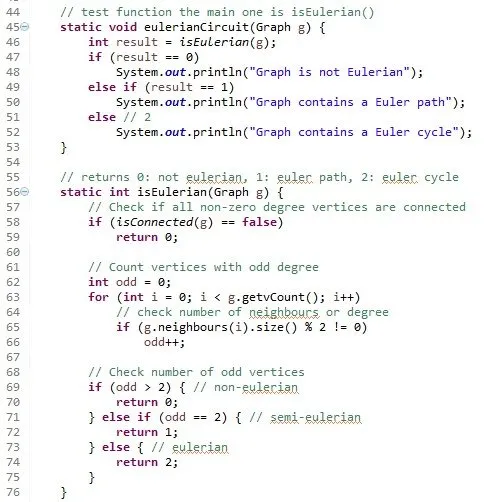Rest of TestGraph that contains the Algorithm: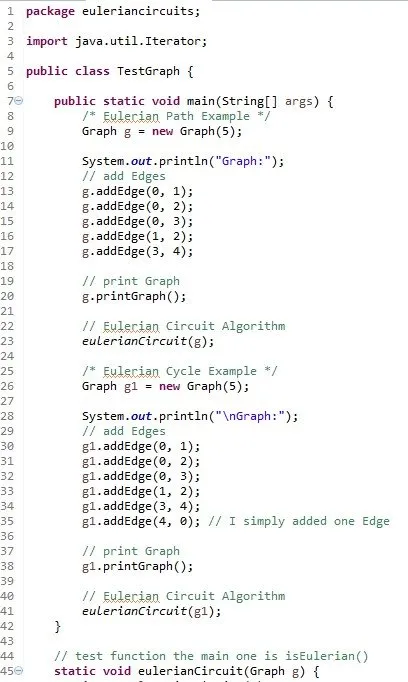Console prints out: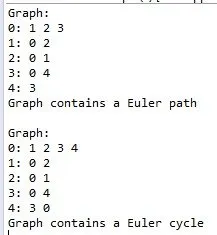If you check closely you will see that these are the Graphs from the examples previously. Only one edge was enough to make the Path a Cycle!

Try running the algorithm for Graphs that doesn't contain a Euler path or cycle!

You can get the code in the following links of pastebin:

Graph class

TestGraph class (that contains the algorithm code)

And this is actually it and I hope you enjoyed it!

Next time in Java Graphs I'm thinking of getting into Boruvka's MST Algorithm and there are even more yet to come! We are actually mostly done with simple stuff and so now only advanced algorithms are left that take more time to be published. But this makes these posts even more interesting!

See ya! Bye!

H2
H3
H4
3 columns
2 columns
1 column
Join the conversation now